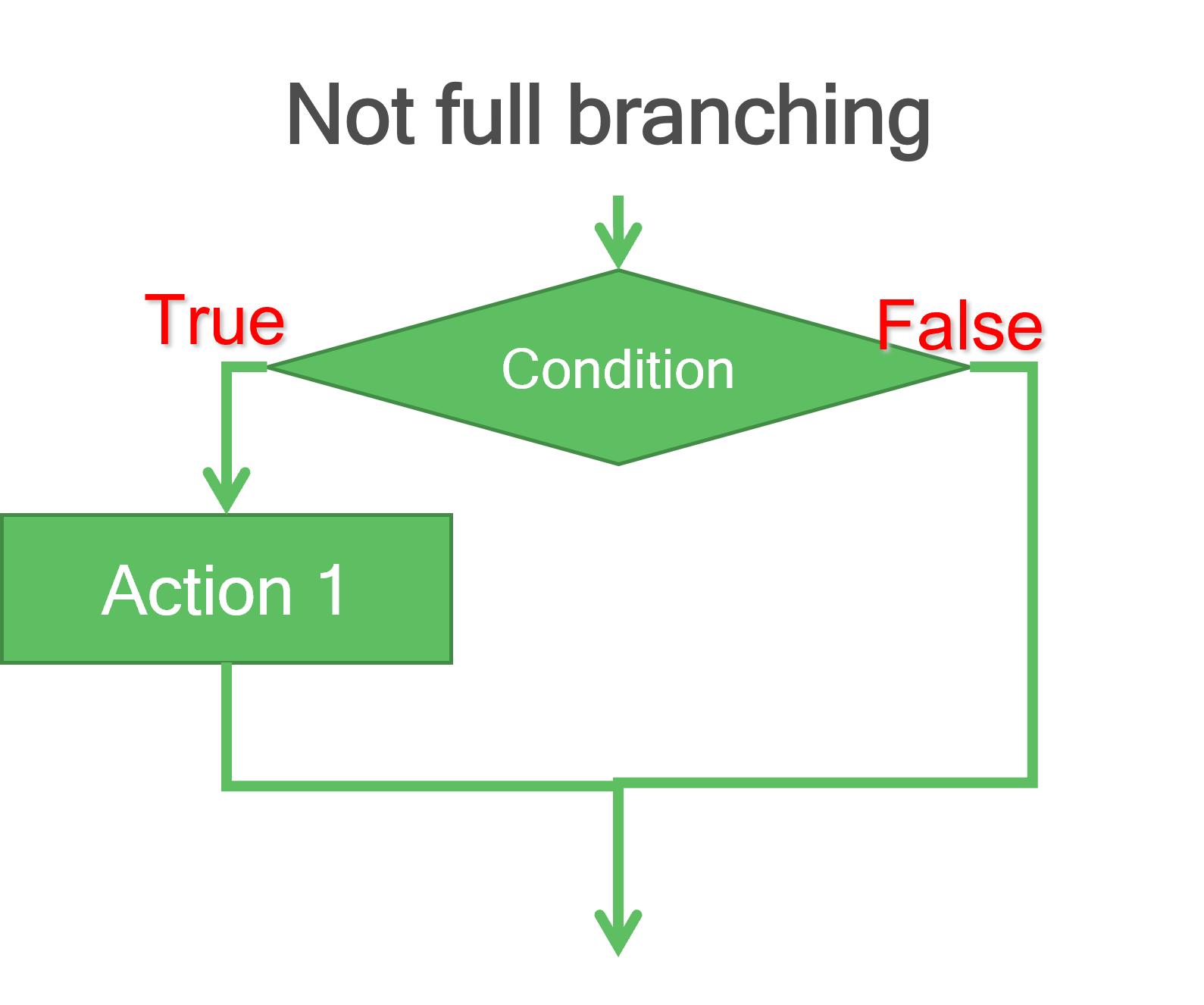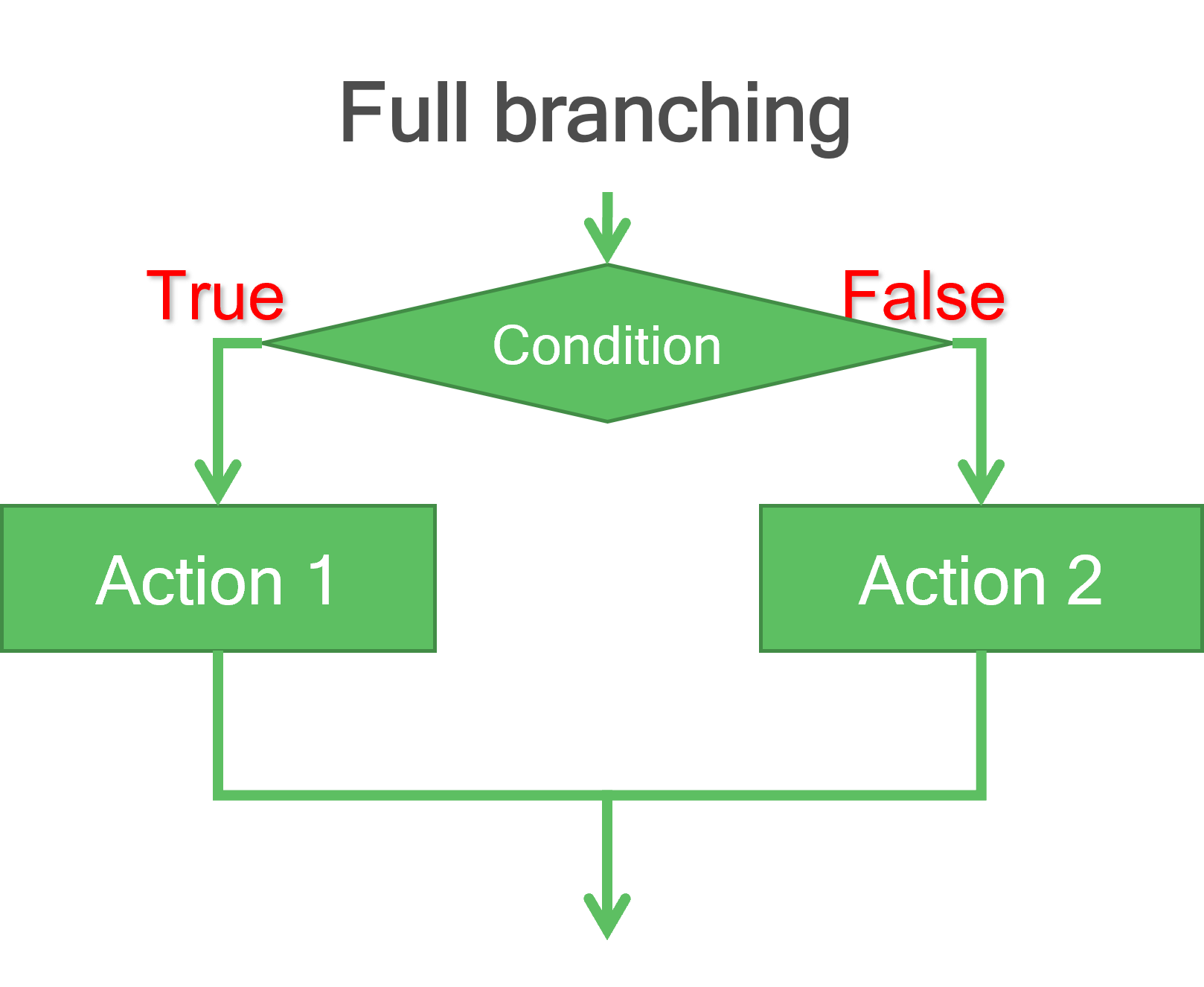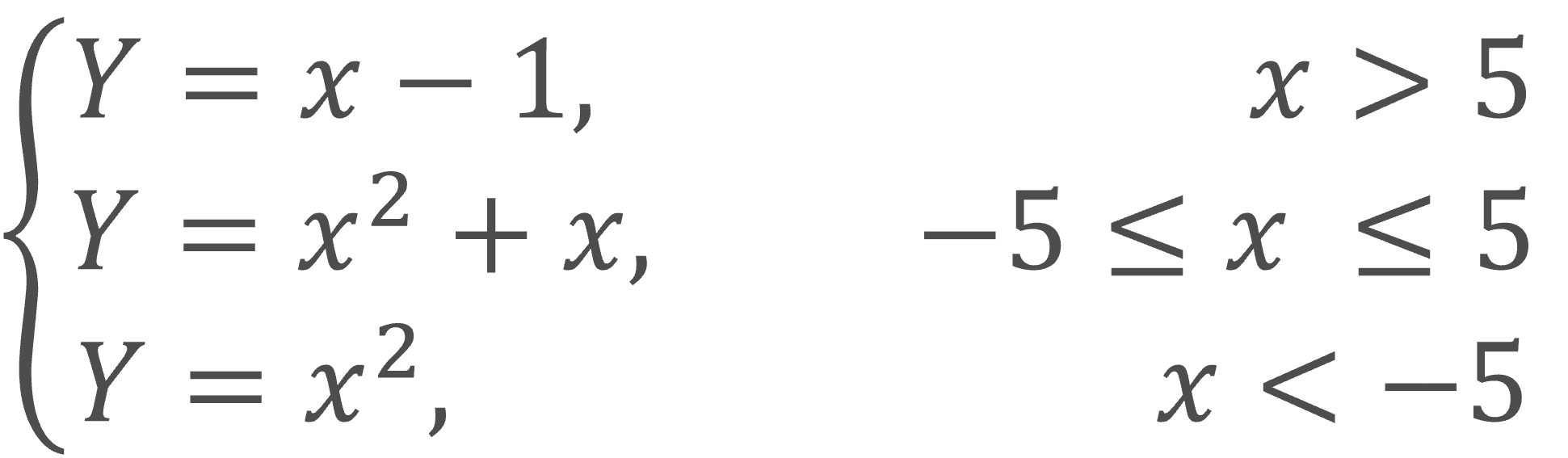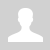Python. If-Else statements

11.1.1.7 apply logic operations to Boolean variables;
11.1.1.9 implement the branching algorithm according to the flowchart;

Python. If-Else statements

Selection - is a programmatic construct in which a piece of code is run only when a condition is met.if :     statement1     statement2     … if :     statement1     statement2     … else:     statement1     statement2     …

Comparison operations

 Operator Meaning == equal > greater < less >= greater or equal <= less or equal != not equal

Example

print('Access is opened!')
else:
print('Error.')Code:

x = float(input())
if x > 0:
Y = 2 * x
else:
Y = x ** 2
print(Y)

One line if statement in Python (ternary conditional operator)

x = int(input("How old are you? "))
print("Kid") if x < 18 else print("Adult")  # ternary conditional operator

x = int(input("How old are you? "))
print("Kid" if x < 18 else "Adult")  # ternary conditional operator

if-elif-else statement

if <bool expression1>:
statement1
…
elif <bool expression2>:
statement1
…
elif <bool expression3>:
statement1
statement2
…
else:
statement1
statement2Code:

x = float(input())
if x > 5:
Y = x – 1
elif x < -5:
Y = x ** 2
else:
Y = x ** 2 + x
print(Y)

Questions:

Exercises:

Категория: Algorithms | Добавил: bzfar77 (12.09.2021)
Просмотров: 259 | | Рейтинг: 5.0/1
 Всего комментариев: 0